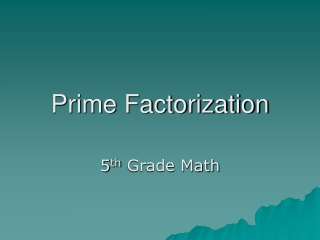DownloadDownload PresentationPrime Factorization

# Prime Factorization

Download Presentation## Prime Factorization

- - - - - - - - - - - - - - - - - - - - - - - - - - - E N D - - - - - - - - - - - - - - - - - - - - - - - - - - -
##### Presentation Transcript

1. Prime Factorization 5th Grade Math

2. Prime Factorization Of a Number • A prime number is a counting number that only has two factors, itself and one. Counting numbers which have more than two factors (such as six, whose factors are 1, 2, 3 and 6), are said to be composite numbers. When a composite number is written as a product of all of its prime factors, we have the prime factorization of the number. • There are several different methods in which can be utilized for the prime factorization of a number.

3. Using Division • Prime factors can be found using division. • Keep dividing until you have all prime numbers. The prime factors of 78 are 2, 3, 13.

4. Remember the Divisibility Rules • If the last digit is even, the number is divisible by 2. • If the last digit is a 5 or a 0, the number is divisible by 5. • If the number ends in 0, it is divisible by 10. • If the sum of the digits is divisible by 3, the number is also. • If the last two digits form a number divisible by 4, the number is also.

5. More divisibility rules… • If the number is divisible by both 3 and 2, it is also divisible by 6. • Take the last digit, double it, and subtract it from the rest of the number; if the answer is divisible by 7 (including 0), then the number is also. • If the last three digits form a number divisible by 8, then the whole number is also divisible by 8. • If the sum of the digits is divisible by 9, the number is also.

6. Using the Factor Tree 78 / \ / \ 2 x 39 / / \ / / \ 2 x 3 x 13

7. 72 / \ 8 x 9 / \ / \ 2 x 4 x 3 x 3 // \ \ \ 2 x 2 x 2 x 3 x 3 Another key idea in writing the prime factorization of a number is an understanding of exponents. An exponent tells how many times the base is used as a factor. 72 = 23 x 32 Exponents

8. Let’s Try a Factor Tree! 84 / \ 2 x 42 / / \ 2 x 2 x 21 / / / \ 2 x 2 3 x 7 What is the final factorization? 22 x 3 x 7 = 84

9. 72 / \ 8 x 9 / \ / \ 2 x 4 x 3 x 3 / \ 2 x 2 x 2 x 3 x 3 72 / \ 2 x 36 / / \ 2 x 2 x 18 / / / \ 2 x 2 x 2 x 9 / / / / \ 2 x 2 x 2 x 3 x 3 Factor Trees do not look the same for the same number, but the final answer is the same.

10. Greatest Common Factors • One method to find greatest common factors is to list the factors of each number. The largest number is the greatest common factor. • Let’s find the factors of 72 and 84. 72 1, 2, 3, 4, 6, 8, 9, 12, 18, 24, 36, 72 84 1, 2, 3, 4, 6, 12, 14, 21, 28, 42, 84

11. 72 / \ 8 x 9 / \ / \ 2 x 4 x 3 x 3 / \ 2 x 2 x 2 x 3 x 3 Take the common prime factors of each number and multiply to find the greatest common factor. 84 / \ 2 x 42 / / \ 2 x 2 x 21 / / / \ 2 x 2 3 x 7 2 x 2 x 3 = 12 Prime Factorization is helpful for finding greatest common factors.

12. Resources • Brain Pop – Prime Factors • Brain Pop - Prime Numbers • Brain Pop - Exponents

13. Standards Checks for Understanding • 0506.2.2    Use the prime factorization of two whole numbers to determine the greatest common factor and the least common multiple. • 0506.2.2    Use the prime factorization of two whole numbers to determine the greatest common factor and the least common multiple. • 0506.2.4    Use divisibility rules to factor numbers. • 0506.2.10  Use exponential notation to represent repeated multiplication of whole numbers. Grade Level Expectations • GLE 0506.2.2    Write natural numbers (to 50) as a product of prime factors and understand that this is unique (apart from order).